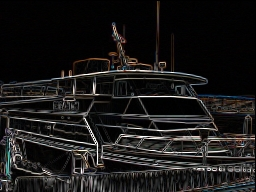# Roberts Edge

The Roberts Edge filter is use to detect edges based applying a horizontal and verticle filter in sequence. Both filters are applied to the image and summed to form the final result. The two filters are basic convolution filters of the form:

Horizontal FilterVerticle Filter
 1 0 0 -1
 0 1 -1 0

For example, if a 2x2 window is used as such

 p1 p2 p3 p4
where the filter is centered on p1 with p2 being pixel[x+1][y] and p3 being pixel[x][y+1], etc. then the forumla to calculate the resulting new p1 pixel is

pixel = abs(p1-p4)+abs(p2-p3)

which is them clamped to the 0-255 range.

Note that the actual forumla uses the horizontal and verticle components into the final form

pixel = SQRT((X*X)+(Y*Y))

where X = abs(p1-p4) and Y = abs(p2-p3)

but for performance reasons we approximate the result and leave the final formula out.

The Roberts Edge detector is fast since the filter is small but it is also subject to interference by noise. If edges are not very sharp the filter will tend not to detect the edge. See Prewitt or Sobel filters instead which are larger and less sensative to noise.

## Example

 Source Roberts Edge